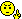# Number of coins

#### marshall1432

##### Banned
Here is my question:

A vending machine only accepts Quarters and Dimes. At the end of a day there are 200 coins in the machine that total $48.05. How many quarters are in the machine and how many dimes? Well first off...I am not good in math so I used my calculator to determine that there are 187 Quarters and 13 Dimes, totaling$48.05.

I am unsure how to set up the equation. Is this anywhere close?

.25x + .10y = 48.05?

ANY HELP WOULD BE APPRECIATED THANKS!

#### o_O

##### Full Member
Yes you're on the right track. Now since you have two variables, it would be pretty difficult to solve for one without any other information. But, you know that there are 200 coins. If x represents your # of quarters and y represents your # of dimes, can you figure out how these relate?

#### Subhotosh Khan

##### Super Moderator
Staff member
marshall1432 said:
Here is my question:

A vending machine only accepts Quarters and Dimes. At the end of a day there are 200 coins in the machine that total $48.05. How many quarters are in the machine and how many dimes? Well first off...I am not good in math so I used my calculator to determine that there are 187 Quarters and 13 Dimes, totaling$48.05.

I am unsure how to set up the equation. Is this anywhere close?

.25x + .10y = 48.05? .....correct

The other equation could be obtained from:

"... there are 200 coins in the machine ..."

ANY HELP WOULD BE APPRECIATED THANKS!

#### marshall1432

##### Banned
No, I am still lost, sorry#### o_O

##### Full Member
All the quarters and dimes you have must add up to how many coins? Then just put that in an equation using your variables x and y.

#### marshall1432

##### Banned
Again I am not good at math??? I am so lost.

#### o_O

##### Full Member
You have x (# of quarters) and y (# of dimes). What must they add up to ?

#### stapel

##### Super Moderator
Staff member
marshall1432 said:
Again I am not good at math??? I am so lost.
When you've posted previously, you've been working with much-more-advanced material. But you showed about as much effort then as you are now. :roll:

If you are honestly incapable of figuring out how to translate "x quarters plus y dimes equals two hundred coins" into an equation, then, once again, I'm afraid you are simply much too "lost" for us to help. You still need to consider hiring a qualified tutor, local to your area, to re-teach you the missing background material, and you may need again to reconsider your choice of courses.

I'm sorry, but we just can't make it any simpler, and we still aren't going to do your homework for you.Eliz.

#### TchrWill

##### Full Member
A vending machine only accepts Quarters and Dimes. At the end of a day there are 200 coins in the machine that total $48.05. How many quarters are in the machine and how many dimes? Well first off...I am not good in math so I used my calculator to determine that there are 187 Quarters and 13 Dimes, totaling$48.05.
I am unsure how to set up the equation. Is this anywhere close?
.25x + .10y = 48.05?

Your equation is correct. While several people have pointed out the second equation that would enable you to solve in the traditional manner, you can proceed to a solution starting with your equation

1--.25Q + .10D = 48.05 or 5Q + 2D = 961
2--Dividing through by the lowest coefficient yields Q + Q/2 = 480 + 1/2
3--(Q - 1)/2 must be an integer k making Q = 2k + 1
4--Substituting back into (1) yields D = 478 - 5k
5--Now, recognizing the fact that the total number of quarters and dimes is 200, you can derive the other equation that people have been trying to convey to you, Q + D = 200. By substitution, we derive 2k + 1 + 478 - 5k = 200 from which k = 93.
6--Therefore, Q = 187 and D = 13
7--.25(187) + .10(13) = 46.75 + 1.30 = $48.05. While this is a path to the solution, by making use of the interim factor of k, you can reach the same solution by simply combining the two basic equations of 25Q + 10D = 4805 and Q + D = 200. I'll leave it for you to solve directly using the basic equations of 25Q + 10D = 4805 and Q + D = 200. I'll leave it to you to follow that path. #### marshall1432 ##### Banned Thanks for the help Will. I also understand that Q + D = 200 I am unsure how you got the k out of that (derived) by means of substitution... #### pka ##### Elite Member marshall1432 said: I also understand that Q + D = 200 $$\displaystyle 10D + 25Q = 4805 \Rightarrow \quad 2D + 5Q = 961$$ divide by 5. Substitute: $$\displaystyle \begin{array}{rcl} 2\left( {200 - Q} \right) + 5Q & = & 961 \\ 400 + 3Q & = & 961 \\ Q & = & 157 \\ \end{array}$$ #### marshall1432 ##### Banned PKA, how can that answer be right. I have 200 coins that equal$48.05. If I take the 157 Q and multiply it by .25 I get $39.25 and assuming the rest is D then that would mean 43 multiplied by .10 is$4.30 for a total of $43.55, not the$48.05 I am trying to figure out. Thanks

Oops, I see where you went wrong. When you subtracted 400 from both sides it left you with 3Q=561......Q=187 not 157. A mistake.

Thanks guys. I understand now.